﻿ Localization of Pilferage of Energy Using PLC Signals for an Unbalanced Distribution SystemPublications are Open
Access in this journal
Article Versions
Export Article
• Normal Style
• MLA Style
• APA Style
• Chicago Style
Research Article
Open Access Peer-reviewed

### Localization of Pilferage of Energy Using PLC Signals for an Unbalanced Distribution System

Dj. Taniguevelane , Dr. K. N. Srinivas
International Transaction of Electrical and Computer Engineers System. 2017, 4(1), 39-48. DOI: 10.12691/iteces-4-1-5
Published online: June 16, 2017

### Abstract

Energy theft is a widespread problem results in loss to the utilities and affects the financial viability of utilities. Hence utilities strive for minimization of theft by carrying out various reform projects. Smart meters, AMI and Theft localization algorithms are some of the tools which will enable them to meet the challenge. Many techniques/algorithms are available for theft localization and each of them requires distinct data such as Historic consumption patterns, Network parameter etc. The effectiveness of theft localization depends on the accuracy of input data. The network parameter data of the service line of the consumers is one of the data required for some of the theft localization algorithms which itself is difficult to measure due to the fact that those are laid underground or inside the buildings. This paper analyses some of the theft detection algorithms and its limitations for applying to the LT distribution network and proposes an algorithm to localize the theft using the distance measurement feature of the PLC signals used in smart meters in addition to the communication function. This paper also analyses the effectiveness of the theft localization using the network data obtained with the proposed algorithm.

### 1. Introduction

Energy theft is a widespread problem faced by the utilities all over the world and is much prevalent in developing countries. The energy theft results in loss to the Utilities and affects the distribution system improvement projects which are aimed to reduce losses, provide quality power supply and not the least, the upgradation of distribution system to meet the load growth.The non-undertaking of distribution improvement projects results in inadequate and irregular supplies of electricity, especially in poor countries. Electricity problem has been ranked as the most important issue which affects the businesses of the Indian industries in the 2006 World Bank Enterprise Survey 1.

The electrical power distribution system is the link that connects the utilities and the consumers in the electricity supply chain. Proper accounting and auditing of the input and output energy in the distribution system is one of the important activity to be undertaken by the Utility to prevent theft, as most of the energy theft are prevalent in the distribution segment. Illegal extraction of energy can be categorized in to following types:

1. Tampering the Energy meter: Consumers deliberately tries to tamper the normal functioning of energy meter so that a lower reading of power use is shown than the actual consumption.

2. Pilferage of energy: Stealing electricity can be arranged by rigging a line from the power source to where it is needed, without the knowledge of utilities or by bribing the officials for not taking any action.

3. Ineffective Billing: Some power organization officials may not be very effective in measuring the amount of electricity used and intentionally or unintentionally can give lower figure than the accurate one.

4. Over draw energy beyond the subsidized limits: Some low income group consumers, where power is supplied without meters, overdraw beyond the eligible subsidized limits.

5. Unpaid bills: Some persons and organizations delay the payment and if it becomes huge amount will desert by winding up their business or will declare as bankrupt.

The first-step in electricity theft reduction is to identify the amount of non-technical losses. The approaches of “100% metering” and “Energy Audit” are some of the measures that can be adopted to arrive at the quantum of non-technical losses. Once the quantum of non-technical losses is arrived, measures to reduce the same could be undertaken. Some of the measures that can be implemented are: (a) providing tamper proof meters, (b) providing meters to unmetered consumers, (c) providing aerial bunched cables in the areas where theft is very high and (d) formation of anti-theft squad and carrying frequent inspection of consumer premises.

The above methods of curbing unauthorized extraction of energy are general measures which invite the manual intervention and the success rate depends on the integrity of the utility personnel. With the advent of smart meters, some of the meter tampering methods that are prevalent in electromechanical meters such as the methods given below could be prevented:

• Disconnecting the neutral wire and using loads with neutral connected to ground.

• Exposing electromechanical meters to magnets.

• Interchanging input and output connections.

Smart meters have the anti-tampering facilities such as to compare phase and neutral current and record the highest of two reading and issue alert by ’Earth Leakage’ indication, alert reverse current and also issue alerts when the meter covers are opened/damaged. By deploying smart meters, even-though the above disputed issues can be minimized, pilferage of energy i.e., taking connections directly (both phase and neutral) from distribution system bypassing of smart meters results in zero reading and note that these pilferage of energy could not be identified by smart meters.

Smart grid is a much generalized word; it includes diverse kind of infrastructures. Advanced Metering Infrastructure (AMI) is one such distribution infrastructure of smart grid and provides bi-directional communication with metering data logged to the Meter Data Acquisition Server (MDAS) without any manual intervention and shall also have remote tripping and closing of loads connected. Further, smart meters can have the facility of Time of Day (TOD) use of energy and thereby can facilitate dynamic billing regime. Due to above advantages of AMI, it is preferred universally, subject to condition that it should not lead incursion on consumer personal data. Apart from the benefit of eliminating manual intervention in billing, AMI could also be used to control pilferage of energy which smart meter cannot do as standalone system.

The illegal tapping or bypassing of meters is difficult to detect as it is not practicable to check the entire consumer wiring from the distribution mains to the meters regularly by the utility personnel 5. If the AMI system is used, various methods of data analytic techniques could be employed to detect/localize such illegal tapping/bypassing of metering circuit using the metered data of MDAS. Several data analytic techniques are available in the literature to localize pilferage of energy and thereby illegal extraction of energy could be prevented. This paper analyses some of the latest techniques available in the literature and suggests a novel methodology of localization of pilferage of energy.

The rest of the paper is organized as follows: In Section II, major previous works on energy theft detection algorithms are discussed. Section III introduces the mathematical models for unbalanced power distribution system and the application of State estimation for the energy theft localization model. In Section IV the proposed energy theft localization algorithm is described in detail. The results of simulations of the proposed methodology is presented in Section V and Section VI concludes the paper.

### 2. Related Works

Several works were undertaken for localization of Non-Technical Loss (NTL) Detection which can be broadly classified into Type I and Type II as defined in 6. Generally, the Type-I techniques require historic energy consumption pattern of consumers and the Type-II techniques require network and real time data to detect the bypassing of meters. The Type-I techniques are useful to detect theft for loads whose consumption pattern is already recorded for a particular consumer whereas Type-II techniques not only useful for detecting such existing loads but also effective for identifying theft/pilferage occurring due to new loads of consumers.

The theft localization algorithm of Type-I techniques employs mathematical models. A new approach of utilizing Autoregressive (AR) technique is proposed in 7 which is based on the advanced signal processing using linear prediction. Consumer data were analyzed using AR model in order to predict the quantity of power consumed within the specified interval and consequently, the results obtained were compared with the actual data recorded against the consumer under study. The model developed was used to predict power consumption at 30 minutes interval ahead. Since the load ahead could be predicted, this could be benchmarked to monitor the power consumed by the consumer such that if the quantity of power consumed varies widely with respect to the predicted load, the consumer is suspected as a potential theft on the distribution network hence electricity theft could be localized. Similar types of mathematical approaches such as Support Vector Machine Linear (SVM-Linear), SVM-Radial Basis Function, Artificial Neural Network Multi-Layer Perceptron’s (ANN-MLP), Optimum Path Forest classifier (OPF) etc., for identification of theft are also presented 8. An improved theft localization method based on cooperative game (CG) based inference mechanism for detecting non-technical loss and power blackout in micro distribution systems using the advanced metering infrastructure has been proposed in 9. In this method, fractional order sprott technique is used to extract fractional order dynamic errors relating to changes in load usages including normal conditions. Electricity fraudulent events for multiple buses are referred as multiple agents. These multiple agents are used in cooperative game based inference mechanism for locating abnormalities.

The issue of privacy control in analyzing the AMI data for theft detection is presented in 10. The model presented in the paper focuses on two of the new services that are enabled by the Advanced Metering infrastructure. Firstly the fine-grained anomaly detection that is possible with the higher sampling rates of smart meter and secondly, the ability to shape the load using the advanced demand-response mechanisms that leverage AMI networks, such as direct-load control. The paper has been divided into two sections, first part deal with a game between the electric utility and the electricity thief by optimizing the data collection interval using Neyman-Pearson theory and Nash equilibrium so as to detect the theft. The remaining part is about the problem of privacy-preserving demand response as a control theory problem for selecting maximum sampling interval for smart meters in order to protect the privacy of consumers while maintaining the desired load shaping properties of demand-response programs. The Type-II algorithms generally leverage the inherent property of voltage drop of a bus when load/current increases for theft localization and some of the methods documented are given below.

A procedure of switching of all nodes remotely and sending a low voltage high frequency signal for calculating the individual branch impedances of the distribution system including the service cables that connect to each consumer/meter and comparing of this calculated value with the value measured during installation of the system for detecting illegal load is proposed in 11. In 12, a method of replacing the non-linear set of equations of the load flow problem with voltage and complex power data by a linear set of equations is presented. The influence constants for each bus due to the power consumed on the voltage profile is calculated for time series which do not have any theft and is optimized with least square solution. These constants will be used for localization of theft on the bus when there is deviation of voltage profile with the calculated value. In 12, the voltage profile along the feeder is estimated based on measurements by the smart meters of the current and power factor of each consumer, and a model of the feeder. This estimated voltage profile and the actual measured profile obtained from meters are compared and based on the deviations, the location of illegal abstraction is determined. In 13, a method to detect bad bus demand data by Distribution State Estimation (DSE) by incorporating irregularities due to meter errors and applying ANOVA for evaluating individual meter anomaly is suggested.

The assumption made in the above Type-II method is that the network parameter data provided is accurate. However, in practical situations particularly in distribution environment, it is difficult to estimate the network parameters accurately. Even, if sincere efforts are taken to measure the individual parameters of the network accurately, the same will be prone to errors imposed by measuring instruments. Further, due to the dynamic nature of the distribution system, the parameters will not be constant and parameters errors will result in incorrect prediction. It is therefore desired that there shall be some scientific method to dynamically measure the network parameter and process it to get accurate data for effective NTL localization algorithm to perform. Even though many network parameter estimations algorithms exist, the non-availability of sufficient measurements such line flows at the two ends of lines in LT distribution network will affect the estimation process. In this paper, a modification of state estimation problem to incorporate distance measurement data using PLC signals and Energy meter measurements of LT distribution network is presented to estimate the network parameters.

### 3. System Model

For the purposes of distribution system power flow studies, we model a radial distribution system as a network of buses connected by distribution lines, transformers to a voltage specified source bus. For instance a four-wire grounded wye overhead distribution line shown in Figure 1 results in a 4×4 impedance matrix.

• Figure 1. Model of the three-phase four-wire distribution line

The corresponding equation is: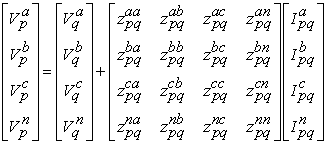(1)

The above equation in matrix form is,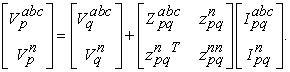(2)

For a grounded neutral, the voltage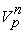and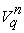can be considered to be equal. In such case, from the first row of eqn. (2), it is possible to obtain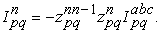(3)

Substituting eqn. (3) into eqn. (2), the final form corresponding to the Kron’s reduction becomes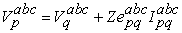(4)

where: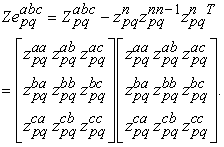(5)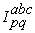is the current vector through the line between bus p and q, can be equal to, the sum of the load currents of all the buses beyond line between bus p and q plus the sum of the charging currents of all the buses beyond line between bus p and q, of each phase.

Therefore the bus q voltage can be computed when the bus p voltage is known, mathematically, by rewriting eqn. (4)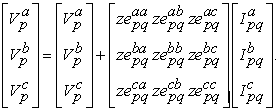(6)

Primitive self-impedance for ‘a’ phase given by Modified Carson equation is: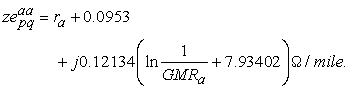(7)

Primitive mutual impedance between ‘a’ and ‘b’ phases given by Modified Carson equation: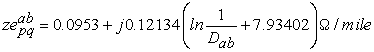(8)

where:

ra is the resistance of conductor ‘a’ in Ω /mile

Ra is Geometric mean radius of conductor ‘a’ in feet

Dab is the distance between conductors ‘a’ and ‘b’ in feet.

Similarly the primitive self and mutual impedances for the other phases could be calculated. Many times the study of power system will use only the positive and zero sequence impedances for the line segments. The methods incorporated for obtaining these impedances is the application of the modified Carson's Equations and the Kron’s reduction. The line-to-ground phase voltages as a function of the line-to-ground sequence voltages are given by: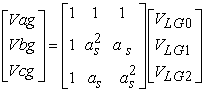(9)

where as is at 120 degree displacements.

In condensed form equation (9) becomes: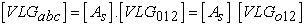(10)

where: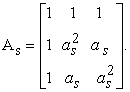(11)

The line current of each phase is defined as: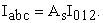(12)

Equation (10) can be used to solve for the sequence line-to-ground voltages as a function of the phase line-to-ground voltages.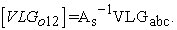(13)

where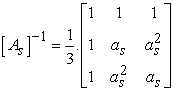(14)

is inverse of As.

Equation (6) can be transformed to the sequence domain by multiplying both sides by As and also substituting in the definition of the phase currents as given by equation (10).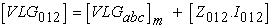(15)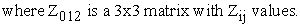(16)

Equation (15) in expanded form is given by: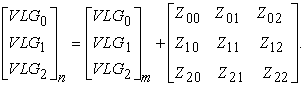(17)

Equation (16) is the defining equation for converting phase impedances to sequence impedances. In equation (16), the diagonal terms of the matrix are the sequence impedances of the line such that: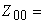Zero sequence impedance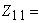Positive sequence impedance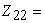Negative sequence impedance

The off-diagonal terms of equation (16) represent the mutual coupling between sequences. In the idealized state, these off-diagonal terms would be zero. In order for this to happen, it must be assumed that the line has been transposed. For high voltage transmission lines, this will generally be the case. When the lines are transposed, the mutual couplings between phases (off-diagonal terms) are equal and consequently the off-diagonal terms of the sequence impedance matrix become zero. As distribution lines are rarely, if ever, transposed, the mutual coupling between phases is not equal and as a result the off-diagonal terms of the sequence impedance matrix will not be zero.

If a line is assumed to be transposed, the phase impedance matrix is modified so the three diagonal terms are equal and all of the off-diagonal terms are equal. The usual procedure is to set the three diagonal terms of the phase impedance matrix equal to the average of the diagonal terms of equation (6) and the off-diagonal terms equal to the average of the off-diagonal terms of equation (6). When this is done, the self and mutual impedances are defined as: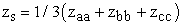(18)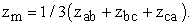(19)

The 3x3 phase impedance matrix Zabc will now have all diagonal elements as zs and all off-diagonal elements as zm. Let this be the equation (20).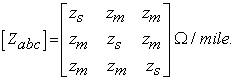(20)

When equation (16) is used with this phase impedance matrix, the resulting sequence matrix is diagonal (off-diagonal terms are zero). The sequence impedances can be determined directly as: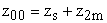(21)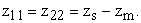(22)

The above positive sequence values are utilized for modified WLS algorithm for pilferage localization proposed in this paper. A working on impedance calculation for 556,500 conductors is furnished in Appendix.

### 4. Proposed Method

In 14, it is shown that the Power Line Carrier (PLC) Communication signals can be used to determine the topology estimation. Further in 15, it is shown that the Power Line Carrier (PLC) Communication signals can be used to predict the distance of conductors used in power systems by measuring the Time of Arrival (ToA) to an accuracy of 30 cm for UWB systems. Suppose Node A sends a message to Node B at time 0 and Node B receives the message at tb. Let the Node B sends a reply packet to A after a known time T. Also let the Node A receives the packet at time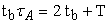. As the time ta is related to the distance d, with c, the speed of light, the distance, d can be derived as,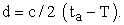With the distance obtained above and using the topography estimated using PLC signals, the R, X and B values can be obtained by knowing the type of conductor and its manufacturing data. The network data so obtained can be used for NTL localization algorithms. However, as errors will be introduced during the PLC distance measurements, the measured data if applied directly will impact the theft localization calculation accuracy. In 16, a method of estimation of network parameters during non-theft hours using PLC signals and the network parameters so obtained for using theft localization has been proposed.

A new method of obtaining much accurate parameter estimation from the measured PLC distance data using modified state estimation algorithm for unbalanced distribution systems is proposed in this paper. The state estimation is traditionally used to evaluate the present state of power system through evaluation of likely V and θ values using weighted least square method with the metered values such as P, Q, I etc., at various node points and with the accurate network parameters. In the above state estimation problem, equations are formed for the measured quantities and partial derivation of the above equations with respect to V & θ are performed along with iterative solution for Taylor series expansion for minimizing the errors so as to obtain the state estimation values. In the new method proposed, equations for the metered values such as P, Q, V, I etc., along with the network parameter obtained from conductor length measured using PLC signal are formed as shown below.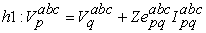(23)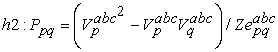(24)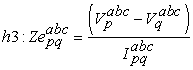(25)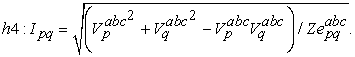(26)

The partial derivatives of the above equations with respect to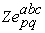are used to form H matrix as given below: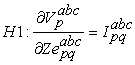(27)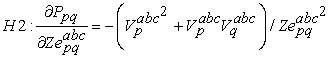(28)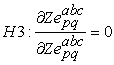(29)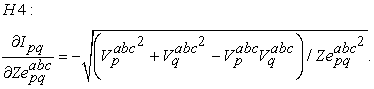(30)

The value of the network parameter is obtained by iterative solution of weighted least square formation: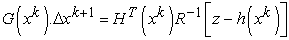(31)

Where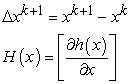R is the variance of each measurement

Z is the measured value.

The WLS estimation is solved to obtain the elements of residual vector and the Largest Normalized Residual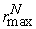for identification of a single bad data existing in the network measurement set is used to localize the theft/NTL.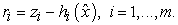(32)

Normalized residual is,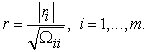(33)

### 5. Results and Analysis

In order to evaluate the proposed algorithm, tests were conducted on modified IEEE 13 bus system and European LV test feeder (Figure 2). It is assumed that measurement of the state equations are available at least on one side of the each bus and the susceptance value is ignored as the same will be negligible in distribution systems. First to get the simulated measured values of the state equations such as Voltage at each bus, Active Power flow and Current in each branch, the unbalanced distribution load flow is performed in the Open DSS software. Random errors that can occur in the measurements are introduced in the results obtained from the load flow output and the simulated PLC measurements for Positive sequence impedance of the network. The above data are fed to the proposed State estimation algorithm developed in MATLAB and the normalised residual errors for impedance are calculated. The bus with the maximum residual error for the impedance would be the bus where theft is identified.

• Figure 2. IEEE 13 bus test feeder

A. Tests on Modified IEEE 13 Bus Feeder

As the objective of the test is to localize the theft for a radial LV distribution network which does not generally have voltage regulators, shunt capacitors, the same are ignored in the simulation tests. Further, the distributed loads are assumed to be concentrated at one third of the line section. For example, the distributed loads as per the IEEE 13 bus test feeder between buses 632 and 671 (Figure 2) are assumed to be concentrated at a fictitious bus 670. The study is conducted for theft simulation for two conditions i.e., theft at one bus and theft at two buses simultaneously. For the first condition, 50% of load as theft is simulated at bus671 with 100% and 50% loading conditions and the residual error generated between the buses 670 and 671 is high and hence the theft is localized for bus 671. Similarly, for the second condition of theft at two buses namely at 671 and 652, the residual error generated for the associated line segments is high and hence the theft is localized for the above two buses.

The results of the proposed deduction of pilferage of energy algorithm are Tabulated in Table 1 and Table 2.

B. TESTS ON EUROPEAN LV TEST FEEDER

The study is also conducted for European LV Test feeder which has 906 buses, as indicated in Figure 3. The test feeder is at the voltage level of 416 V (phase-to-phase), which is typical in the European low voltage distribution systems. Theft are simulated at bus 12 with 50% of load as theft and the residual error for impedance measurement obtained for the associated line segment is found to be high amongst all and hence the theft is localized for bus 12. Table 3 shows the results of simulation.

• Figure 3. Single-line diagram of the European low voltage test feeder

The results show that the theft can be localized with the proposed methodology of obtaining normalized residual error for PLC distance measurement for feeder lengths. In respect of the applicability of the methodology for low loading conditions, it is shown that theft pilferage up to 25% of bus load during 50% of loading of distribution systems could be detected.

### 6. Conclusion

This paper presents a new algorithm to tackle one of the major threats of electric power utility, called the energy theft. Methodology of obtaining normalized residual error for PLC distance measurement for feeder lengths has been proposed for the first time. This novel method to detect pilferage of energy using the feeder length measurements using Time of Arrival of PLC signals, can minimize the problem of obtaining network parameter data of a distribution network for energy pilferage localization. This method will be a new addition in the literature of deduction of pilferage of electric energy.

The results show that the theft can be localized with the proposed algorithm. In respect of the applicability of the methodology for low loading conditions, it is shown that theft pilferage up to 25% of bus load during 50% of loading of distribution systems could be detected.

The validation of the proposed algorithm has been implemented considering a Numerical example relating to impedance calculations for unbalanced systems (IEEE-13 bus 601 line configuration) using Kron’s reduction Technique, which is presented in Appendix 1.

Future studies can be made to maximize the accuracy of the pilferage detections and also for micro-grids having distribution generation integration so that the power utilities could be benefited.

### References

  Miriam Golden and Brian Min, “Theft and Loss of Electricity in an Indian State”, Working paper, IGC International Growth Centre, 2012. In article View Article  Thomas B. Smith, “Electricity theft: a comparative analysis,” Energy Policy, vol. 32, no. 18, pp. 2067-2076, 2004. In article View Article  Shantanu Dixit, Girish Sant,SubodhWagle and N. Sreekumar,”HT Energy Audit: The Crucial Starting Point for Curbing Revenue Loss” Prayas Occasional Report, February 2002. In article  M. Anas, N. Javid, A. Mahmood, S. M. Raza, U. Qasim, A. Khan, “Minimizing Electricity Theft using Smart Meters in AMI”, arXiv, 11 August 2012. In article View Article  K. N. Srinivas and D. Taniguevelane, “Analysis of Smart meter data forEnergy Pilferage localization Techniques”, IEEE International Conference on Standards for Smart Grid Ecosystem, 6-7 March 2014, Bangalore. In article  V. J. Hodge and J. Austin “A survey of outlier detection methodologies” Artificial Intelligence Review, vol. 22, pp. 85-126, 2004. In article View Article  A.I. Abdullateef, M.J. E. Salami, M.A. Musse, A.M. Aibinu, and M.A. Onasany, “Electricity Theft Prediction on Low Voltage Distribution System Using Autoregressive Techniques”, International Journal of Research in Engineering and Technology (IJRET) Vol.1, No.5, 2012 pp. 250-254. In article View Article  Anas M, Javaid N, Mahmood A, Raza SM, Qasim U, Khan ZA., “Minimizing electricity theft using smart meters”, in AMI; 2012. In article View Article  Tung-Shen Zhan, Shi-Jaw Chen, Chih-Cheng Kao, Chao-Lin Kuo, Jian-Liun Chen, Chia-Hung Lin, “Non-Technical loss and power blackout detection under advanced metering infrastructure using a cooperative game based inference mechanism”, IET Generation, Transmission & Distribution, 2016, Vol.10, Iss. 4, pp. 873-882. In article View Article  Alvaro A. Cardenas University of Texas,Dallas. Saurabh Amin, MIT. Galina Schwartz, Roy Dong, Shankar Sastry, University of California, Berkeley. “A Game Theory Model for Electricity Theft Detection and Privacy-Aware Control in AMI Systems”, IEEE 50th Annual Allerton Conference Communication, Control, and Computing (Allerton), 2012. In article View Article  A. Pasdar, S. Mirzakuchaki, “A Solution to Remote Detecting of Illegal Electricity Usage Based on Smart Metering”, 2nd IEEE International Workshop on Soft Computing Applications, 21-23 August, 2007. In article View Article  SamWeckx, Carlos Gonzalez, JeroenTant, Tom De Rybel, Johan Driesen, “Parameter Identification of Unknown Radial Grids for Theft Detection”, 2012 3rd IEEE PES Innovative Smart Grid Technologies Europe (ISGT Europe), October 14-17, 2012. In article View Article  Frank Van Den Bergh, Petr Kadurek, Sjef Cobben and Wilkling, “Electricity Theft Localization based on Smart Metering”, CIRED 21st International Conference on Electricity Distribution, paper no. 1267. In article  Shih-Che Huan, Yuan-Liang Lo, and Chan-Nan Lu, “Non-Technical Loss Detection Using State Estimation and Analysis of Variance”, IEEET ransactions on Power Systems, Vol.28. No.3, August 2013. In article View Article  TomasoErseghe, Francesco Lorenzon, Stefano Tomasin, AlessandroCostabeber and Paolo Tenti, “Distance Measurement over PLC for Dynamic Grid Mapping of Smart Micro Grids” IEEE SmartGridComm, 2011. In article View Article  Tomaso Erseghe, Stefano Tomasin and Alberto Vigato, “Topology Estimation for Smart Micro Grids via Power line Communications”, IEEE Transactions on Signal Processing, Vol.61, No.13, July 1, 2013. In article View Article  Vijay Arya and Balakrishnan Narayanaswamy, “Loss localisation in smart distribution networks”, 2014 Sixth International Conference on Communication Systems and Networks (COMSNETS), 6-10 Jan. 2014. In article View Article  Muhammad Tariq, H. Vincent Poor, “Electricity Theft Detection and Localization in Grid-tied Microgrids”, IEEE Transactions on Smart Grid, Volume: PP, Issue: 99, 25 August 2016. In article View Article  Luís Marques; Nuno Silva; Ismael Miranda; Eduardo Rodriges; Helder Leite, “Detection and localisation of non-technical losses in low voltage distribution networks”, Conference on Power Generation, Transmission, Distribution and Energy Conversion, Nov, 2016. In article View Article  Yuchen Zhou, Xiaodao Chen, Albert Y. Zomaya, Lizhe Wang and Shiyan Hu, “A Dynamic Programming Algorithm for Leveraging Probabilistic Detection of Energy Theft in Smart Home”, IEEE Transactions on Emerging Topics in Computing,Vol.3, Dec’15. In article View Article  K. N. Srinivas and D. Taniguevelane, “Deduction of Pilferage of Energy using PLC signals”, International Journal of Applied Engineering Research, Vol. 10, No. 9, 2015. In article

### APPENDIX

Numerical example relating to impedance calculations for unbalanced systems (IEEE-13 bus 601 line configuration) using Kron’s reduction Technique:

1. The conductor data, conductor spacing’s used for IEEE 13 bus system are given hereunder

• Figure 4. Impedance calculation using Kron’s reduction

1: Conductor size in AWG

2: Type of conductor: AA = All Aluminum

ACSR = Aluminium Conductor Steel Reinforced

CU = Copper

3: 60 Hz resistance at 50 degrees C (Ohms / mile)

4: Conductor outside diameter (inches)

6: Amp Capacity at 50 degree C.

2. Based on the above conductor data, the GMR distance and resistance values obtained from col. No 5 and 3 of above Table 4 respectively are given below:

3. The inter conductor spacing’s for the distribution overhead line configuration ID-500 obtained from Fig 1 are given below:

Dab = 2.5 ft. Dbc = 4.5 ft. Dca = 7 ft

4. The conductor spacing’s which are not provided exclusively for phase to neutral are calculated as per normal mathematic equation gives the following values:

Dan = 5.6569 ft. Dbn = 4.272 ft. Dcn = 5 ft

5. The self impedance is calculated as per the below formula: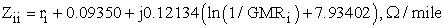6. Accordingly the first row and first column of Z matrix is calculated as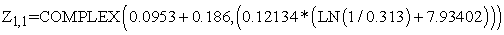and is given in Table 5 below.

7. The mutual impedance between the conductor is calculated as per below formula: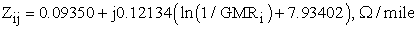8. Accordingly the first row and second column is calculated as: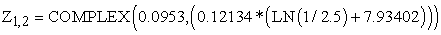9. Kron’s reduction assumes that neutral of each bus is considered to be earthed. Hence the above equation Zaa (4 x 4 matrixes) can be reduced as 3x3 matrix as per the formula given below: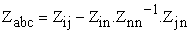where: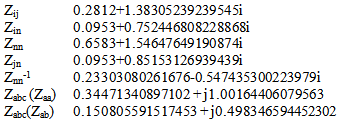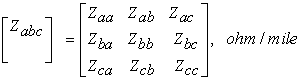The self and mutual impedance i calculated as:

z1 = 1/3 (zaa + zbb + zcc), Ω/mile

z2 = (0.3447 + j1.002 + 0.3447 + j1.002 + 0.3447 + j1.002) / 3

z3 = 0.3447 + j 1.002

zm = (0.1508 + j 0.4983 + 0.1508 + j 0.4983 + 0.1508 + j 0.4983) / 3

The phase impedance matrix is now defined as:The sequence impedances can be determined directly as: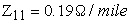The above positive impedance is calculated for each line of the distribution system and the impedance matrix for whole distribution system is formed.

The distribution system can be analyzed with the above calculated positive sequence impedance using the following formula: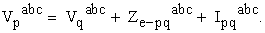This work is licensed under a Creative Commons Attribution 4.0 International License. To view a copy of this license, visit http://creativecommons.org/licenses/by/4.0/